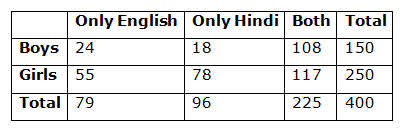# CWC/FCI Prelims 2019 – Quantitative Aptitude Questions (Day-27)

Dear Readers, Exam Race for the Year 2019 has already started, To enrich your preparation here we are providing new series of Practice Questions on Quantitative Aptitude – Section for CWC/FCI Exam. Aspirants, practice these questions on a regular basis to improve your score in aptitude section. Start your effective preparation from the right beginning to get success in upcoming CWC/FCI Exam.

[WpProQuiz 5719]

Directions (1 – 5): What value should come in the place of question mark (?) in the following questions?

1) (32 % of 7500) ÷? = 24 % of 2500

a) 6

b) 9

c) 4

d) 7

e) None of these

2) (4/21) of 10605 + (4/13) of 7150 =? × 4

a) 1055

b) 920

c) 840

d) 725

e) None of these

3) [(1/29) × 4350] + (5/13) × 2470 =? × 5

a) 360

b) 440

c) 300

d) 220

e) 280

4) 3 1/7 + 7 5/14 – 8 3/7+ 2 9/14 =? – 3 ½

a) 8 (3/14)

b) 8 (3/5)

c) 9 (1/3)

d) 6 (5/6)

e) None of these

5) (5/11) of (7/9) of 2475 – ? + (4572 ÷ 4) = 463

a) 1280

b) 1555

c) 1370

d) 1490

e) None of these

Directions (6 – 10): These questions are based on the following data. Study it carefully and answer the questions that follow.

In a school having 400 students, boys and girls are in the ratio of 3:5. The students speak Hindi, English or both the languages. 12% of the boys speak only Hindi. 22% of the girls speak only English. 24% of the total students speak only Hindi and the number of boys speaking both the languages is six times the number of boys speaking only Hindi.

6) How many boys speak Hindi?

a) 18

b) 126

c) 108

d) 26

e) None of these

7) How many girls speak only Hindi?

a) 55

b) 117

c) 96

d) 78

e) None of these

8) How many students speak English?

a) 304

b) 79

c) 225

d) 117

e) None of these

9) The number of girls speaking only Hindi is what per cent of the total number of students speaking only Hindi?

a) 38.2%

b) 71.8%

c) 31.2%

d) 78%

e) 81.25%

10) What is the ratio of the number of boys to the number of girls speaking both the languages?

a) 23:25

b) 12:25

c) 12:13

d) 25:13

e) 13:25

Direction (1-5) :

[(32/100)*7500] ÷ x = (24/100)*2500

X = 4

(4/21)*10605 + (4/13) *7150 = 4x

2020 + 2200 = 4x

4220 = 4x

X = 4220/4 = 1055

[(1/29) × 4350] + (5/13) × 2470 = 5x

150 + 950 = 5x

5x = 1100

X = 1100/5 = 220

3 1/7 + 7 5/14 – 8 3/7+ 2 9/14 + 3 ½ = x

X = (3 + 7 – 8 + 2 + 3) (1/7 + 5/14 – 3/7 + 9/14 + ½)

X = 7[(2 + 5 – 6 + 9 +7)/14]

X = 7(17/14) = 8(3/14)

(5/11) * (7/9) * 2475 – x + (4572/4) = 463

875 – x + 1143 = 463

875 + 1143 – 463 = x

X = 1555

Directions (6 – 10):

Number of boys = 3/8 * 400 = 150 and number of girls = 250

Boys who speak only Hindi = 0.12 * 150 = 18

Girls who speak only English = 0.22 * 250 = 55

Students who speak only Hindi = 0.24 * 400 = 96

Hence Girls who speak only Hindi = 96 – 18 = 78

Hence Girls who speak both languages = 250 – 55 – 78 = 117

Boys who speak both languages = 18*6 = 108

Hence Boys who speak only English = 150 – 18 – 108 = 24No. of boys who speak Hindi = 18 + 108 = 126

78 girls speak only Hindi

No. of students speaking English = 400-18 -78 = 304

Required % = 78/96 * 100 = 81.25 %